## Example Questions

### Example Question #1 : Mixed / Improper Fractionsandare positive integers.is a multiple of.

Quantity A: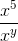Quantity B: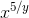The relationship cannot be determined.

Quantity A is greater.

The two quantities are equal.

Quantity B is greater.

The relationship cannot be determined.

Explanation:

Recall that the exponent of the denominator is sutracted from the exponent of the numerator.

Therefore Quantity A is equivalent to x5–y, and because we would then be comparing an arithmetic operation to a geometric operation, it does not matter whether y is a multiple of 5.  The two quantities cannot be compared.

### Example Question #7 : Mixed / Improper Fractions

Which of the following is the mixed fraction equivalent to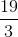?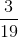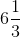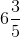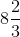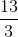Explanation:

To begin, notice that using your calculator, you can find: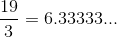Now, the closest even multiple ofthat is less thanis.  Therefore, you know that your number is: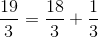This is the same as: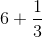, or simply,.  This is your mixed fraction.

### Example Question #8 : Mixed / Improper Fractions

Which of the following is equivalent to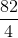?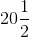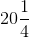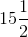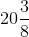Explanation:

Although there are many ways to convert improper fractions into mixed fractions, the easiest way is to use your calculator to your advantage.  Begin by dividing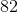by.  This gives you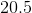. Therefore, you can eliminate all the options that have do not havefor their first portion. Next, multiplyby the denominator (), and get.  This means that you haveand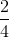, or.  Thus, your answer is.

### Example Question #2 : Mixed / Improper Fractions

Quantity A: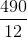Quantity B: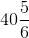Which of the following is true?

The two quantities are equal

The relationship of the two quantities cannot be determined based on the information provided.

Quantity B is larger.

Quantity A is larger.

The two quantities are equal

Explanation:

Though there are several ways you could solve this, let's convert the improper fraction into a mixed one so we can compare them. Start by dividing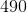by.  This gives you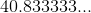Now, since we know that the two numbers have the same whole-number value, we need to compare their decimal portions. Compare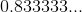to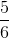. The latter is indeedTherefore, the two values are equal.

### Example Question #1 : Mixed / Improper Fractions

Write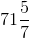as an improper fraction.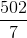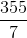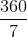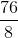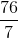Explanation:

To find the improper fraction value, we must effectively add together 71 and 5/7. To do this, we will give 71 a denominator of 7; therefore, we are transforming 71/1 to x/7.  The shortest way to do this is to multiply by 7/7 (which really is 1); therefore, 71 = 71 * (7/7) = 497/7.

Now add them: (497 + 5)/7 = 502/7

### Example Question #3 : Mixed / Improper Fractions

Which of the following improper fractions is equivalent to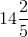?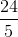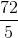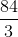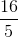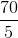Explanation:

To find an improper fraction, you need to multiply the whole number that you have by the denominator of the associated fraction.  For our problem, this means that you will multiplyby, getting.  Next, you add this to the numerator of your fraction, giving you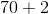, or.  Finally, you place this over your original denominator, giving you:### Example Question #4 : Mixed / Improper Fractions

Which of the following improper fractions is equivalent to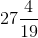?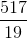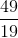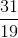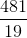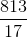Explanation:

To find an improper fraction, you need to multiply the whole number that you have by the denominator of the associated fraction.  For our problem, this means that you will multiplyby, getting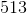.  Next, you add this to the numerator of your fraction, giving you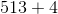, or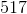.  Finally, you place this over your original denominator, giving you:Tired of practice problems?

Try live online GRE prep today.